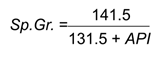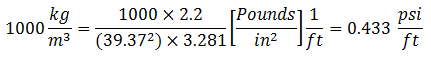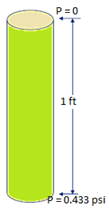## Specific Gravity & API Gravity

A specific gravity scale developed by the American Petroleum Institute (API) for measuring the relative density of various petroleum liquids, expressed in degrees. API gravity is graduated in degrees on a hydrometer instrument and was designed so that most values would fall between 10° and 70° API gravity. The arbitrary formula used to obtain this effect is presented in the following paragraphs.

Specific Gravity (SG)

Specific gravity is a dimensionless value that compares all liquids to fresh water and all gases to air.  Certain liquid physical values can be calculated by multiplying the specific gravity of that liquid by the value for fresh water. For gases, the values are calculated by multiplying the specific gravity of the gas by the value for air. Freshwater and air have a specific gravity of one. Liquids and gases with a specific gravity greater than one are heavier than water or air.

API GravityOil density is often reported in terms of API gravity. API gravity can be converted to specific gravity using the API to Specific Gravity Conversion equation, while specific gravity can be converted to API gravity using the Specific to API Gravity Conversion equation. Both equations are presented below:

API to Specific Gravity Conversion:Specific to API Gravity Conversion:### Pressure and depth:

“Pressure and Depth” is the FUNDAMENTAL relationship in the oil industry. Your understanding of the concept is crucial. The easiest way to calculate pressure from depth is to use the pressure gradient of the given fluid.

Pressure gradients for incompressible fluids have units of pressure/depth. For example, psi/ft, bar/m.

Pressure gradient seems difficult, but it is simply using the density of the fluid and converting units:

The density of pure water is 1000 kg/m3. To convert to gradient:• 1 kg = 2.2 pounds
• 1 m = 39.37 inches
• 1 m = 3.28 feet0.433 is the gradient for pure water (SG = 1) in Imperial units, remember it.

NB: Specific Gravity is always relative to pure water.